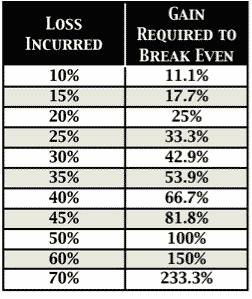September 17, 2021

# Risk of Ruin CalculatorThe Risk of Ruin (Also called the RoR) is a statistical model in trading which quantifies the probability a trader will eventually blow up and lose all of the trading capital in their account. It measures the risk of ruin based on the metrics of a trader or systems win/loss percentage and the percent of capital exposed to loss for each trade. The risk of ruin for a trader or system is one of the most important statistics to be aware of to understand the chances of losing your whole account eventually if you trade big enough for a long enough time. Ruin happens during long losing streaks as capital is destroyed.

The risk of ruin depends on win rate and risk per trade.

Three key questions must be answered.

The win rate is the expectancy of how many times you will have winning trades based on backtesting of a system or your historical record if you are a discretionary trader.

Your potential for risk of loss per trade is based on your position sizing and your stop loss placement. It is measured by a percent of total trading capital at risk. If you are trading a \$100,000 account, with \$10,000 position sizing, and a 10% stop loss on each trade then your risk of loss per trade is a maximum of 1% of total trading capital or \$100,000 / \$1,000 = 1%.

The longest losing streak projection is based on the odds you will eventually lose money in over and over again in a row over a large sample size of trades considering your win rate. This is called a drawdown.

The smaller the percentage of your trading capital you risk on any one trade the lower the risk of ruin.

The odds are that a trading strategy or system will eventually have a 60% win rate over a sequence of 100 trades and then see a losing streak of 10 trades in a row.Probability of a losing streak based on win rate.

So the most important answer for any trader is “Will you survive a streak of 10 losing trades in a row?”

Below are the drawdowns of different risk% of total trading capital starting with a \$100,000 account.The drawdown in capital after 10 losses in a row of different total account risk%.

Keep in mind the returns needed on total trading capital after a drawdown just to get back to even.From our look at the math above to avoid  the risk of ruin it is good policy to only risk 1% to 2% of total trading capital per trade. (This is NOT position size this is risk based on stop loss placement combined with position sizing). Also a win rate of at least 50% to 60% helps limit losing streaks to a maximum of about 10 losses in a row over the long term.

Here are two formulas for calculating risk or ruin probabilities.

Risk of Ruin = ((1 – (W – L)) / (1 + (W – L)))U

W = The probability of a win.

L = The probability of a loss.

U = The maximum number of risks that can be taken before the trader reaches their threshold for ruin.

Here is another formula by Perry Kaufman:

risk_of_ruin = ((1 – Edge)/(1 + Edge)) ^ Capital_Units